#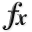1-D Test Functions¶

class go_benchmark.Problem02(dimensions=1)

Univariate Problem02 test objective function.

This class defines the Univariate Problem02 global optimization problem. This is a multimodal minimization problem defined as follows: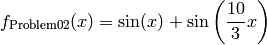Bound constraints: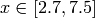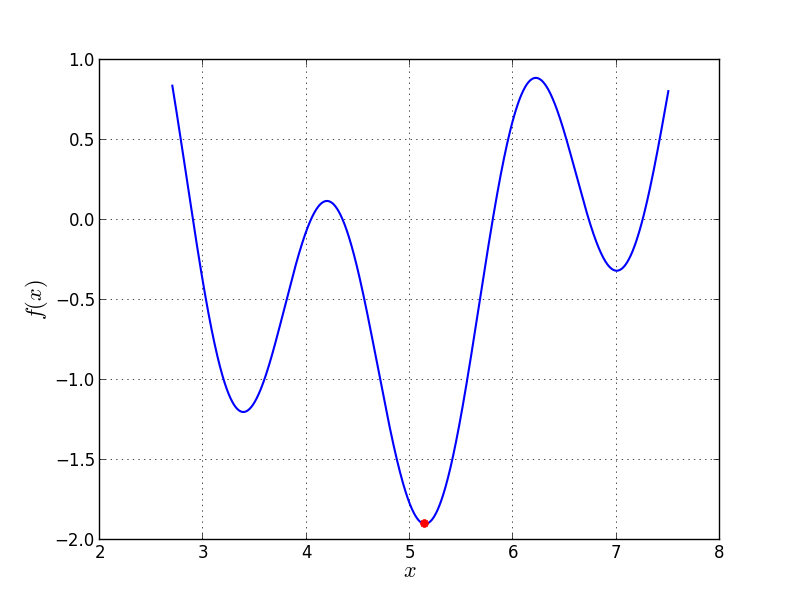Univariate Problem02 function

Global optimum:for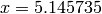class go_benchmark.Problem03(dimensions=1)

Univariate Problem03 test objective function.

This class defines the Univariate Problem03 global optimization problem. This is a multimodal minimization problem defined as follows: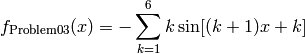Bound constraints: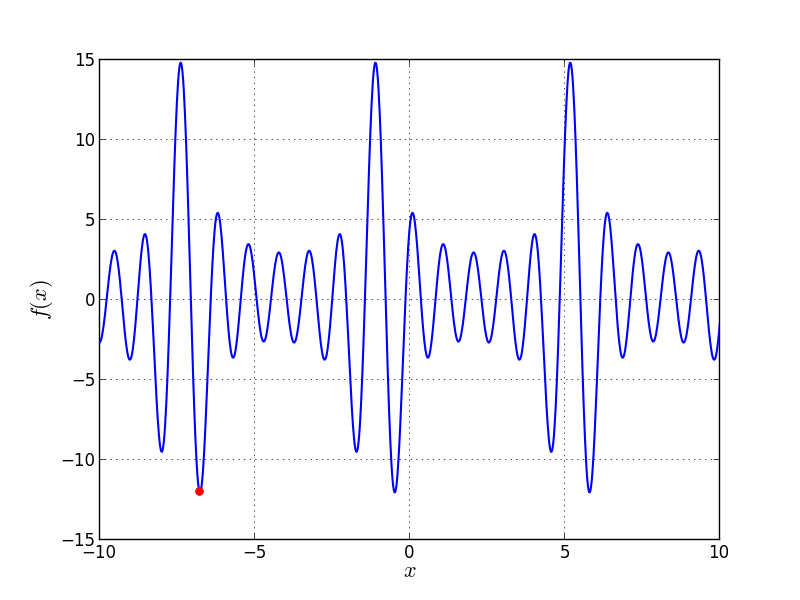Univariate Problem03 function

Global optimum: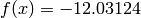for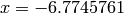class go_benchmark.Problem04(dimensions=1)

Univariate Problem04 test objective function.

This class defines the Univariate Problem04 global optimization problem. This is a multimodal minimization problem defined as follows: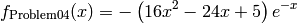Bound constraints: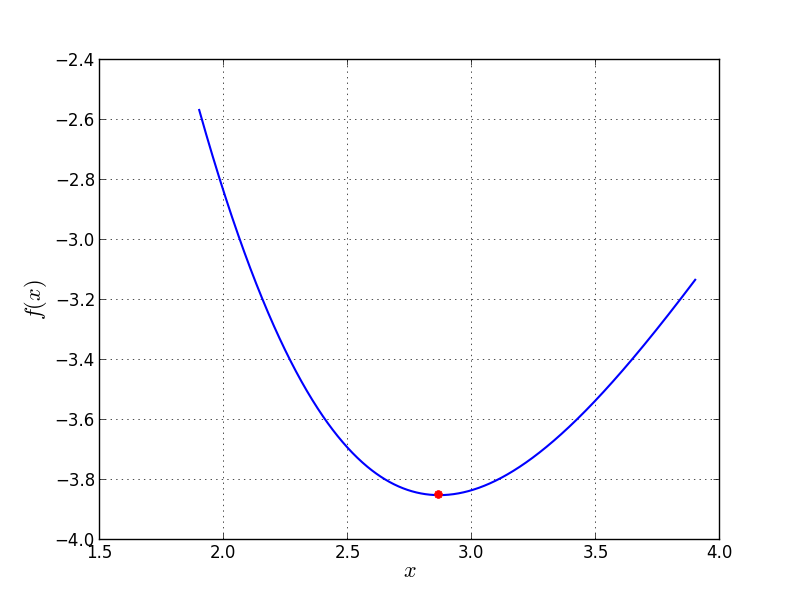Univariate Problem04 function

Global optimum: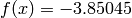forclass go_benchmark.Problem05(dimensions=1)

Univariate Problem05 test objective function.

This class defines the Univariate Problem05 global optimization problem. This is a multimodal minimization problem defined as follows: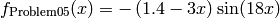Bound constraints: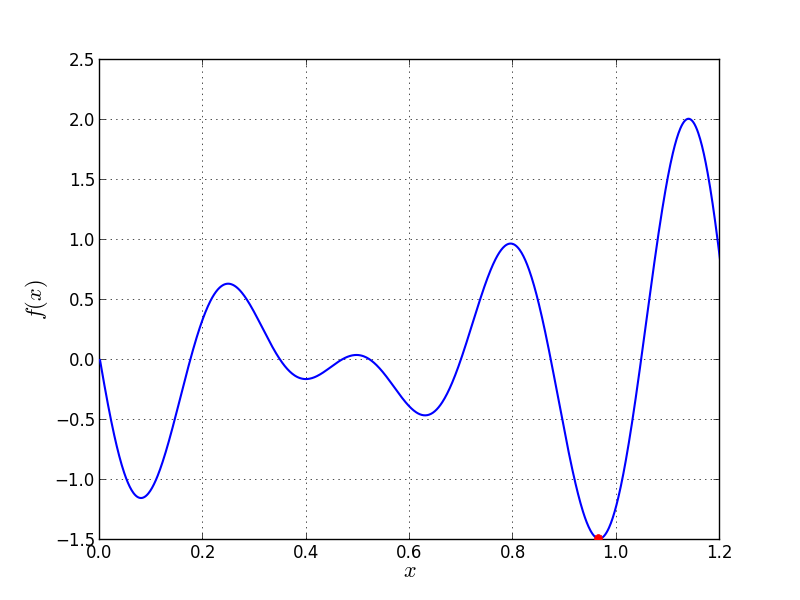Univariate Problem05 function

Global optimum: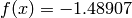for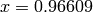class go_benchmark.Problem06(dimensions=1)

Univariate Problem06 test objective function.

This class defines the Univariate Problem06 global optimization problem. This is a multimodal minimization problem defined as follows: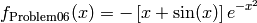Bound constraints: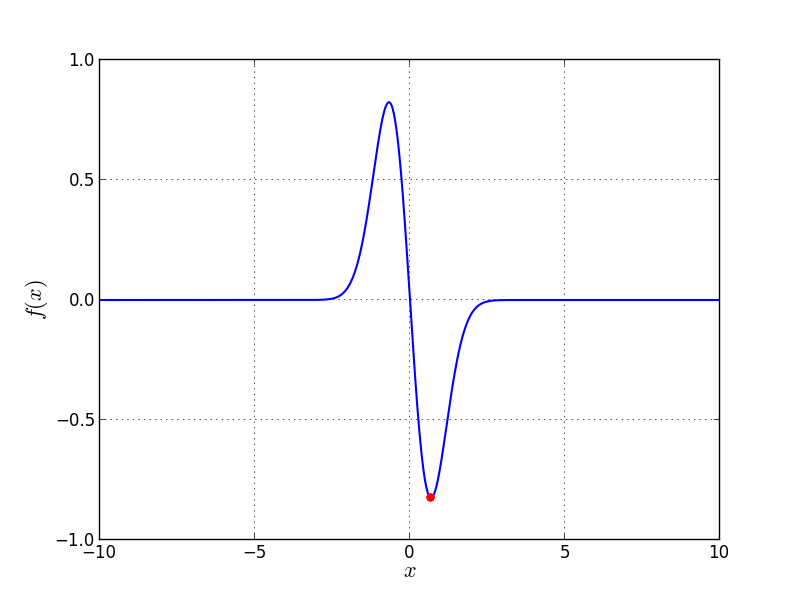Univariate Problem06 function

Global optimum: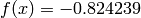for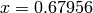class go_benchmark.Problem07(dimensions=1)

Univariate Problem07 test objective function.

This class defines the Univariate Problem07 global optimization problem. This is a multimodal minimization problem defined as follows: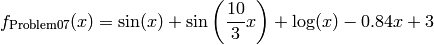Bound constraints: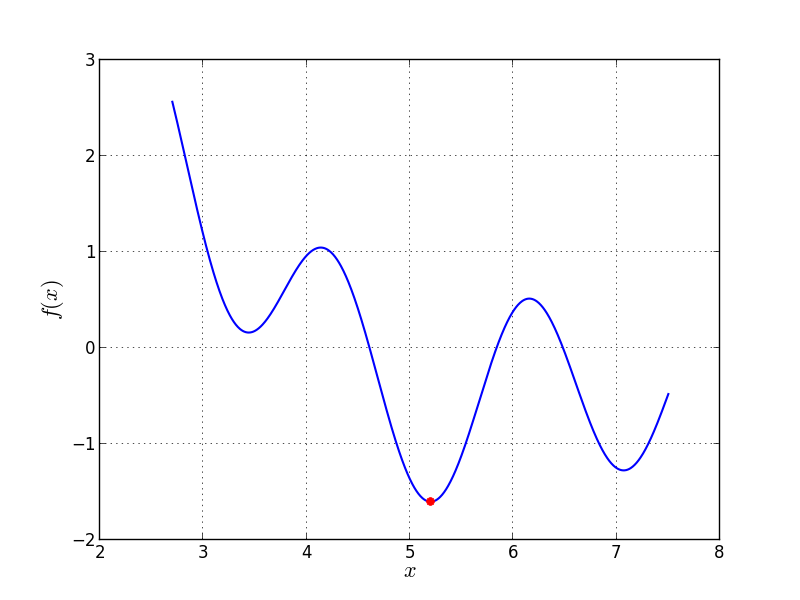Univariate Problem07 function

Global optimum: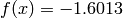for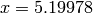class go_benchmark.Problem08(dimensions=1)

Univariate Problem08 test objective function.

This class defines the Univariate Problem08 global optimization problem. This is a multimodal minimization problem defined as follows: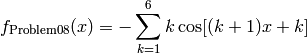Bound constraints: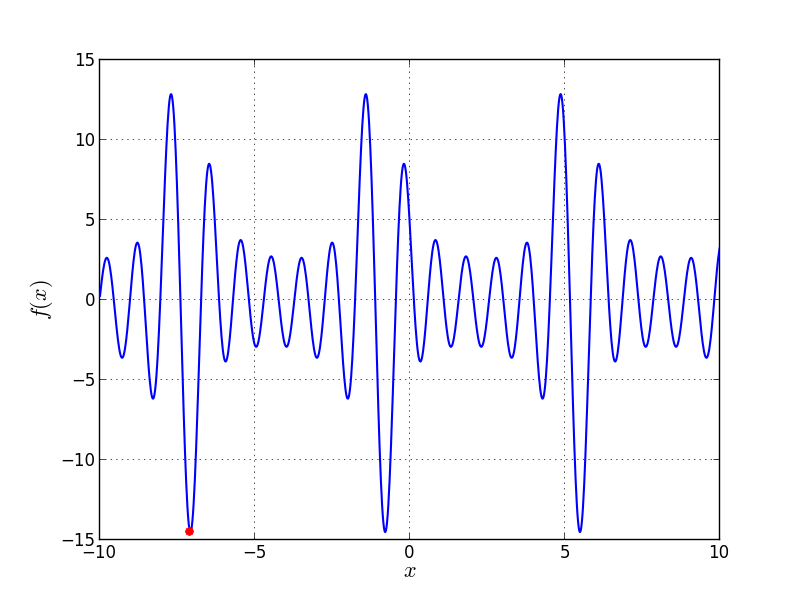Univariate Problem08 function

Global optimum: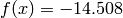forclass go_benchmark.Problem09(dimensions=1)

Univariate Problem09 test objective function.

This class defines the Univariate Problem09 global optimization problem. This is a multimodal minimization problem defined as follows:Bound constraints: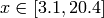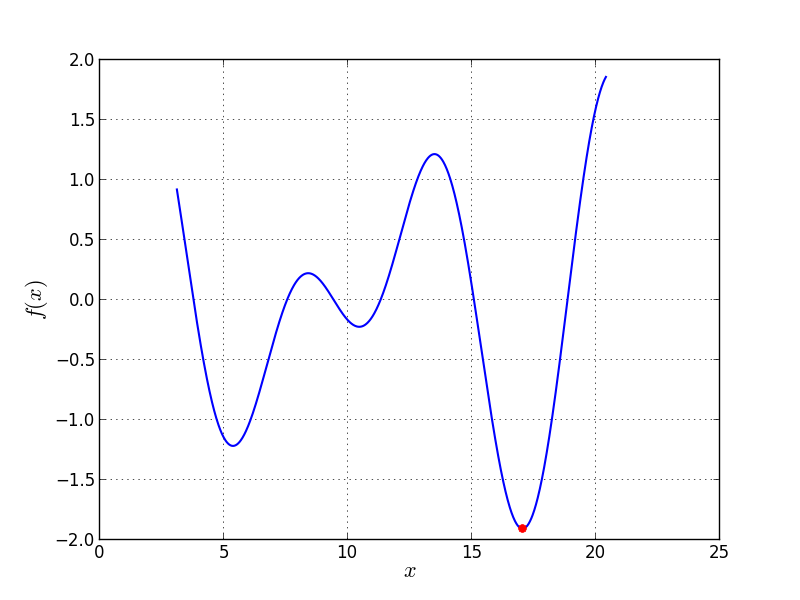Univariate Problem09 function

Global optimum:forclass go_benchmark.Problem10(dimensions=1)

Univariate Problem10 test objective function.

This class defines the Univariate Problem10 global optimization problem. This is a multimodal minimization problem defined as follows: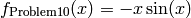Bound constraints: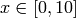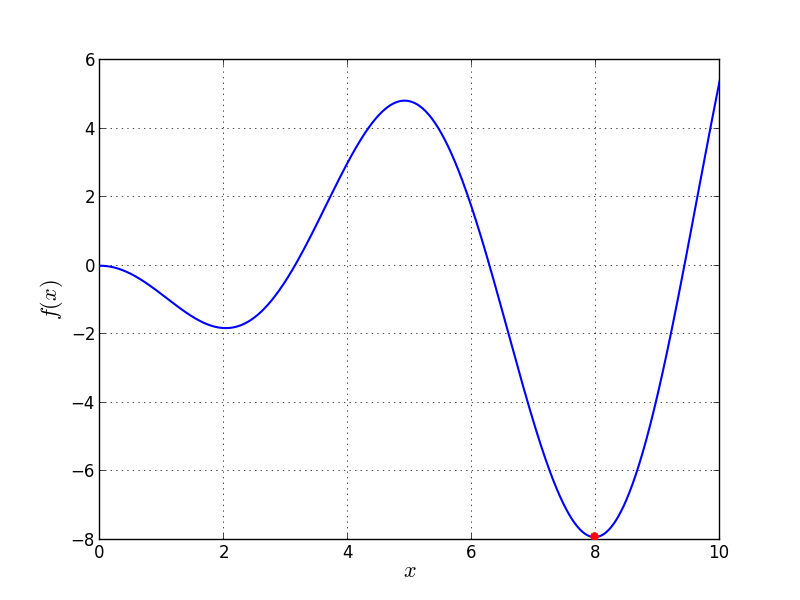Univariate Problem10 function

Global optimum: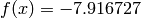for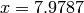class go_benchmark.Problem11(dimensions=1)

Univariate Problem11 test objective function.

This class defines the Univariate Problem11 global optimization problem. This is a multimodal minimization problem defined as follows:Bound constraints: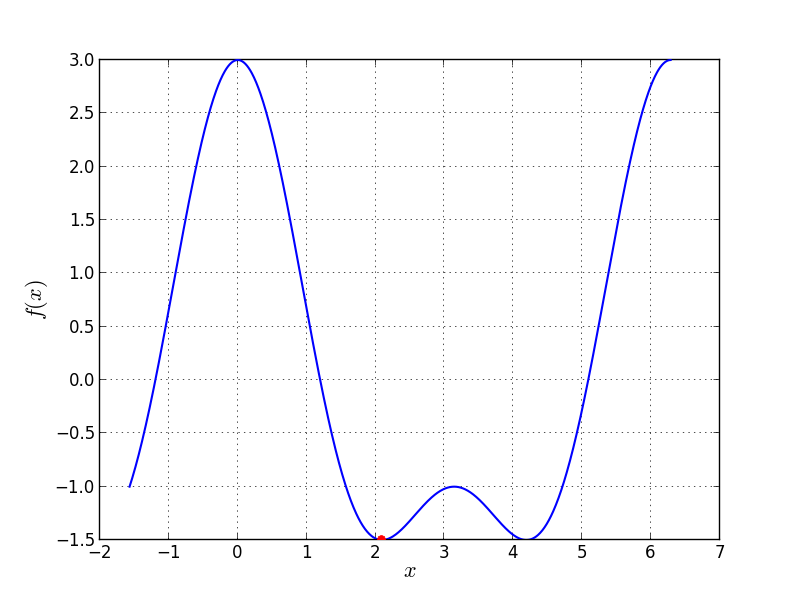Univariate Problem11 function

Global optimum: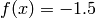for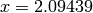class go_benchmark.Problem12(dimensions=1)

Univariate Problem12 test objective function.

This class defines the Univariate Problem12 global optimization problem. This is a multimodal minimization problem defined as follows: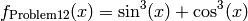Bound constraints: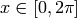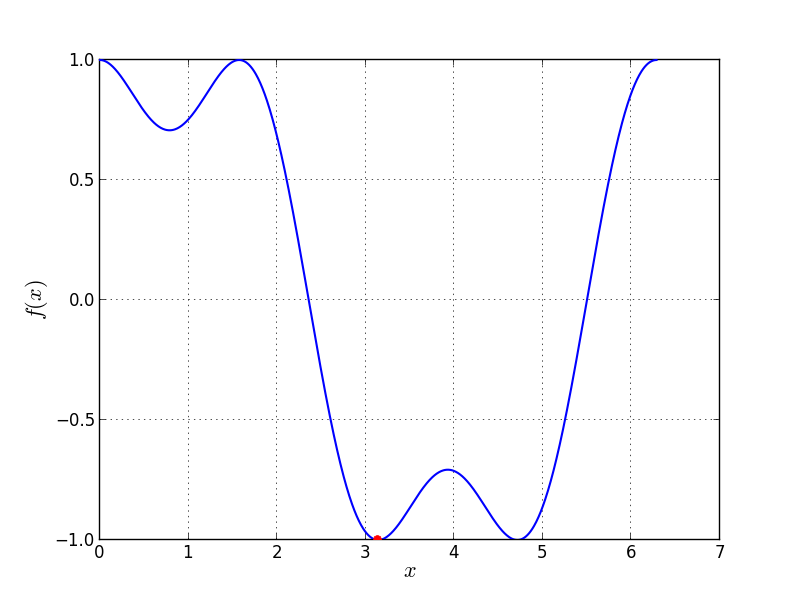Univariate Problem12 function

Global optimum: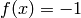for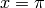class go_benchmark.Problem13(dimensions=1)

Univariate Problem13 test objective function.

This class defines the Univariate Problem13 global optimization problem. This is a multimodal minimization problem defined as follows: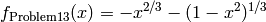Bound constraints: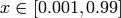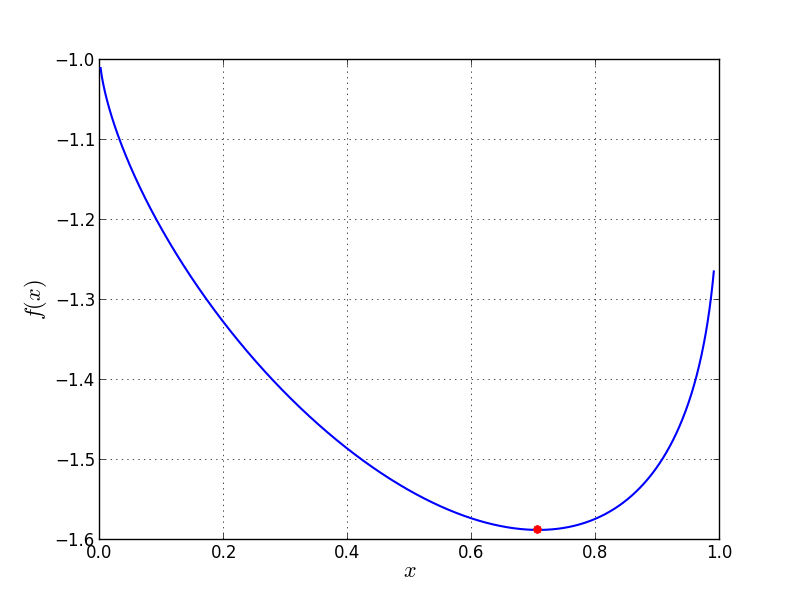Univariate Problem13 function

Global optimum:for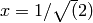class go_benchmark.Problem14(dimensions=1)

Univariate Problem14 test objective function.

This class defines the Univariate Problem14 global optimization problem. This is a multimodal minimization problem defined as follows: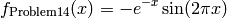Bound constraints: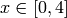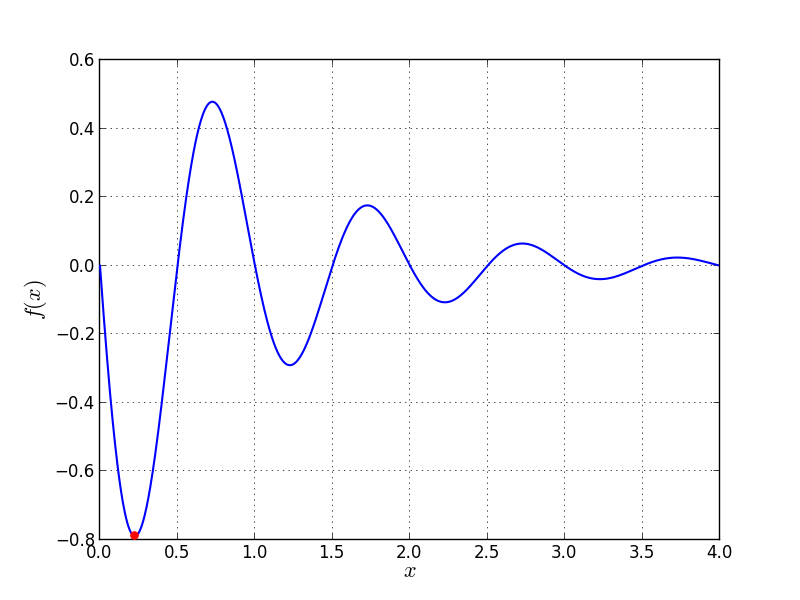Univariate Problem14 function

Global optimum: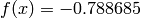forclass go_benchmark.Problem15(dimensions=1)

Univariate Problem15 test objective function.

This class defines the Univariate Problem15 global optimization problem. This is a multimodal minimization problem defined as follows: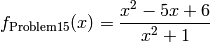Bound constraints: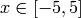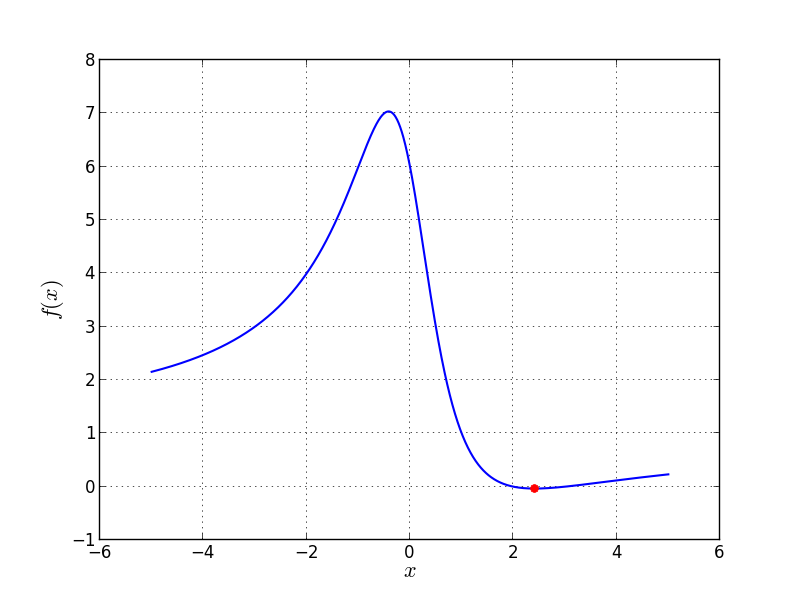Univariate Problem15 function

Global optimum:forclass go_benchmark.Problem18(dimensions=1)

Univariate Problem18 test objective function.

This class defines the Univariate Problem18 global optimization problem. This is a multimodal minimization problem defined as follows: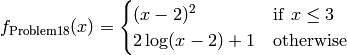Bound constraints: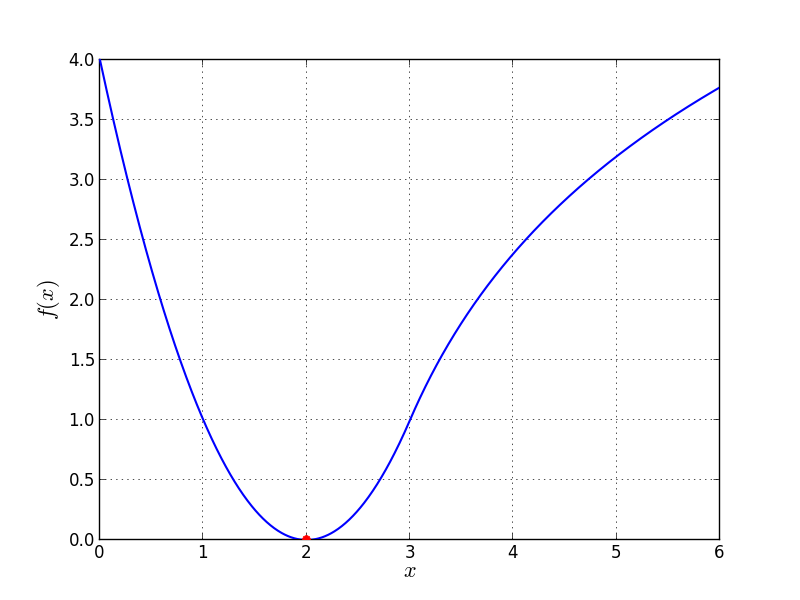Univariate Problem18 function

Global optimum:for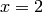class go_benchmark.Problem20(dimensions=1)

Univariate Problem20 test objective function.

This class defines the Univariate Problem20 global optimization problem. This is a multimodal minimization problem defined as follows:Bound constraints: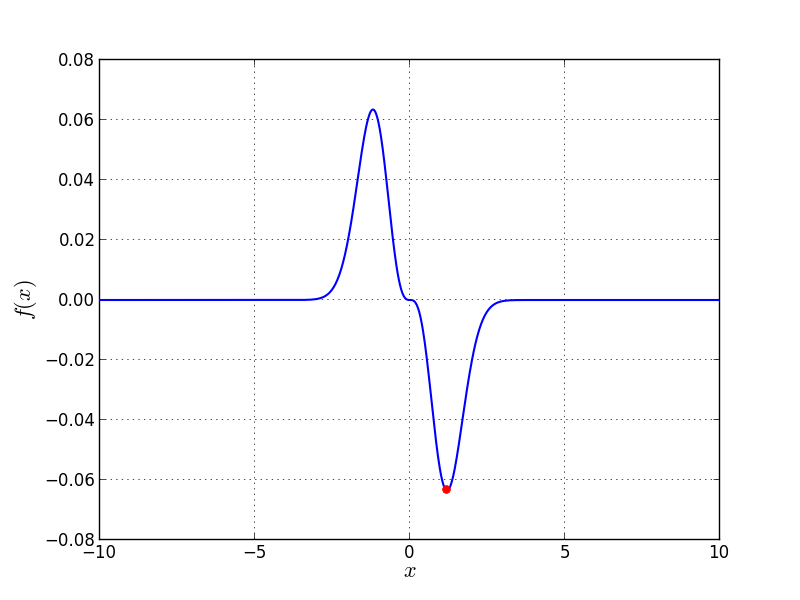Univariate Problem20 function

Global optimum: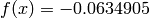for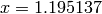class go_benchmark.Problem21(dimensions=1)

Univariate Problem21 test objective function.

This class defines the Univariate Problem21 global optimization problem. This is a multimodal minimization problem defined as follows: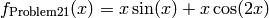Bound constraints: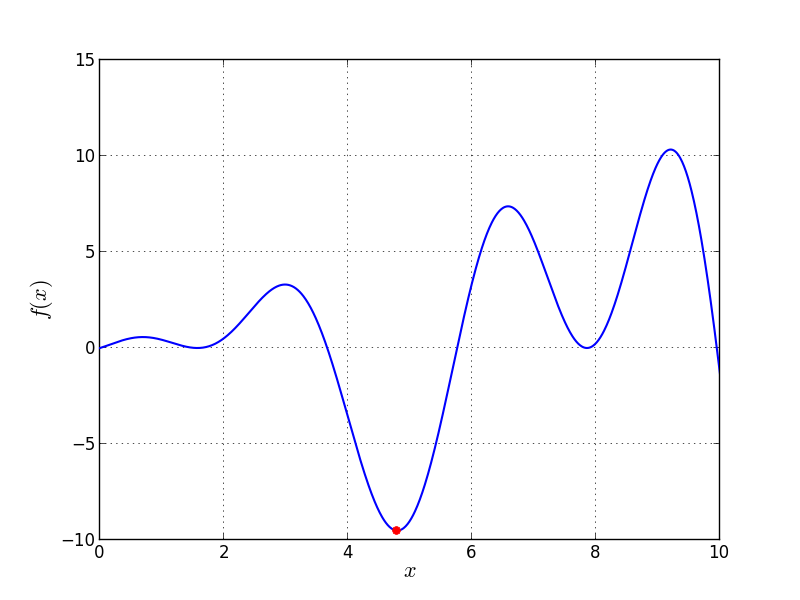Univariate Problem21 function

Global optimum:forclass go_benchmark.Problem22(dimensions=1)

Univariate Problem22 test objective function.

This class defines the Univariate Problem22 global optimization problem. This is a multimodal minimization problem defined as follows:Bound constraints: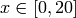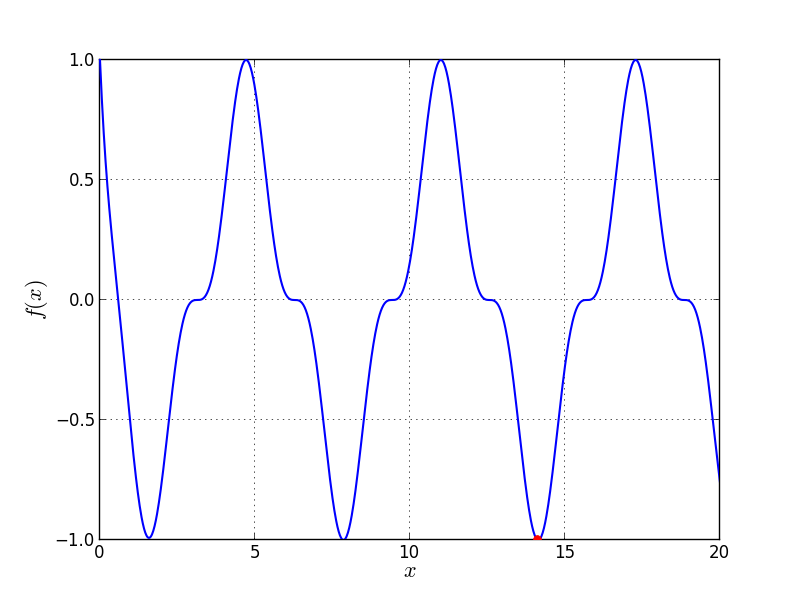Univariate Problem22 function

Global optimum: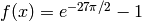for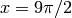#### Previous topic

N-D Test Functions Z